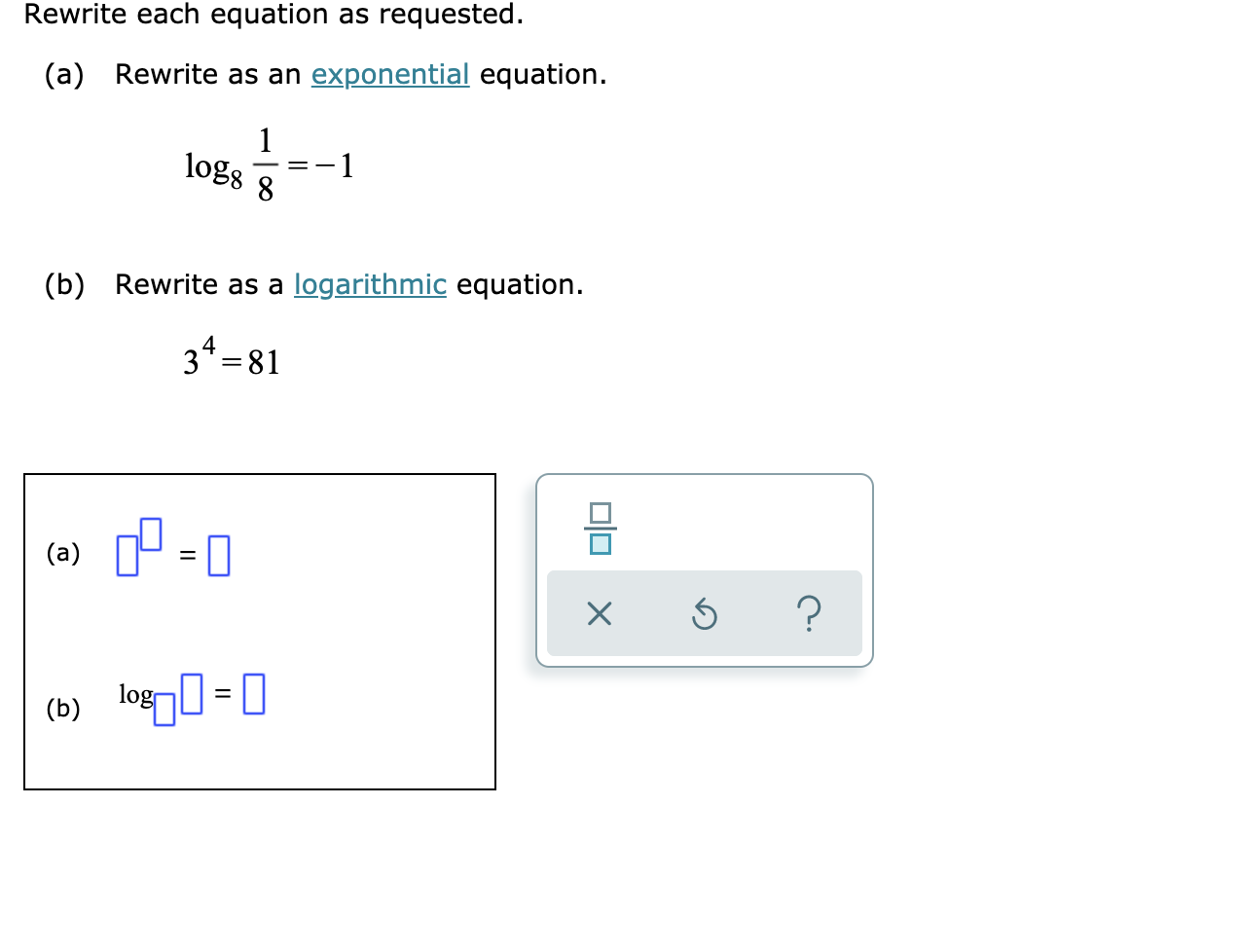# Rewrite each equation as requested.(a) Rewrite as an exponential equation.1log81logarithmic equation(b) Rewrite as a34 81(a)log = ||(b)X

Question
4 views

See attachedhelp_outlineImage TranscriptioncloseRewrite each equation as requested. (a) Rewrite as an exponential equation. 1 log8 1 logarithmic equation (b) Rewrite as a 34 81 (a) log = || (b) X fullscreen
check_circle

Step 1

(a) Rewrite the given equation as an exponential equation as,

Step 2

(b) Rewrite the given equation as ...

### Want to see the full answer?

See Solution

#### Want to see this answer and more?

Solutions are written by subject experts who are available 24/7. Questions are typically answered within 1 hour.*

See Solution
*Response times may vary by subject and question.
Tagged in

### Other